# AP Chemistry : Structure of Ionic Solids

## Example Questions

### Example Question #71 : Compounds And Molecules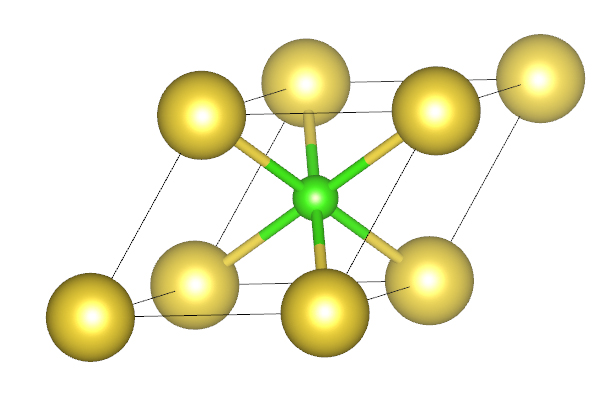How many yellow spheres and green spheres are there per unit cell shown?

8 yellow, 1 green

2 yellow, 1 green

4 yellow, 1 green

1 yellow, 1 green

None of the above

1 yellow, 1 green

Explanation:

For a unit cell, the corners count as 1/8 of a sphere and atoms completely within the unit cell count as 1.  This gives us 1 yellow and 1 green sphere in the unit cell shown above.

### Example Question #2 : Structure Of Ionic Solids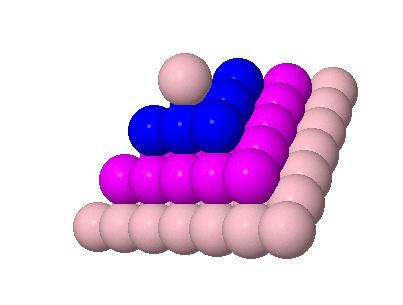Based upon the above image, what type of packing is this?

Tetragonal close packing (tcp)

Simple cubic packing (scp)

Hexagonal close packing (hcp)

Cubic close packing (ccp)

Body centered packing (bcp)

Cubic close packing (ccp)

Explanation:

The figure above represents ABCABC packing which is cubic close packing.

### Example Question #2 : Structure Of Ionic Solids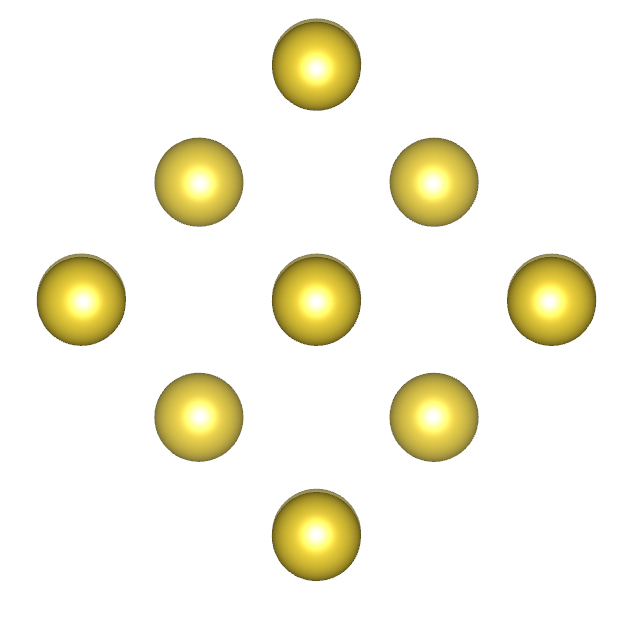Based upon the above image, what type of packing is this?

Tetragonal close packing (tcp)

Body centered packing (bcp)

Hexagonal close packing (hcp)

Cubic close packing (ccp)

Simple cubic packing (scp)

Hexagonal close packing (hcp)

Explanation:

The figure above represents ABABA packing which is hexagonal close packing.

### Example Question #621 : Ap Chemistry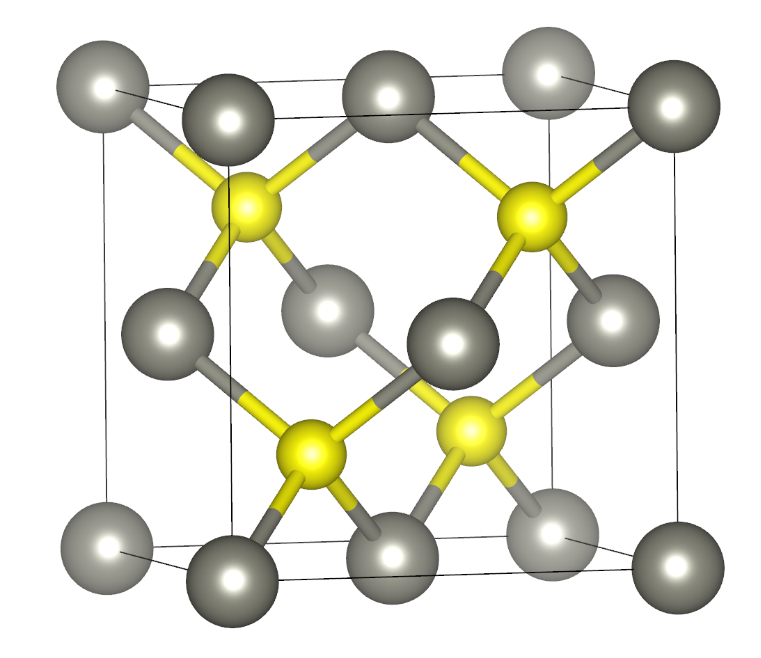Zinc Sulfide can be described as a cubic close packed arrangement of Zn with sulfur occupying one-half of the tetrahedral holes.  Based upon that description and the figure above, what is the formula for zinc sulfide?

Zn3S

Zn12S4

ZnS2

Zn2S

ZnS

ZnS

Explanation:

The figure above has 8 Zn atoms on the corners (counting as 1/8) and 6 Zn atoms on the faces (counting as ½).  This gives 4 Zn atoms in the unit cell.  The Sulfur atoms are completely within the unit cell and count as 1 each for a total of 4 S atoms.  This gives the formula Zn4S4 which reduces down to ZnS.

### Example Question #1 : Structure Of Ionic Solids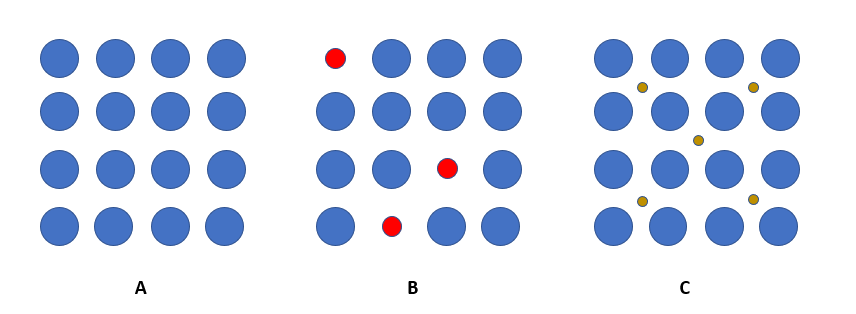What are the names of the alloy types shown in the figure above:

A = Pure Meta; B = Substitutional; C = Interstitial

A = Interstitial; B = Pure metal; C = Substitutional

A = Pure Metal; B = Interstitial; C = Substitutional

A = Substitutional; B = Interstitial; C = Pure Metal

None of the above